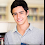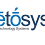How to convert lat long DD to DMS coordinates Convert lat and long from DD coordinates, using Google Maps API.

# How to convert lat long DD to DMS coordinates

by April 26, 2014

The geographical coordinates used by Google Maps are designated as coordinates DD (Decimal Degrees). In other words, these coordinates are in Decimal Degrees format (e.g. Lat 40.601203, Lng -8.668173). Another representation format of geographic coordinates, quite common among GPS users, is the DMS format (Degrees, Minutes, Seconds, e.g N 40° 36' 4.3", W 8° 40' 5.4").
From DD coordinates, used to insert markers on the map, you can easily convert lat and long coordinates to DMS format. For sure this information will be very useful to your visitors.

You may also be interested in:
Geographic coordinates converter

### The geographic coordinate system

It should first be noted that both systems divide the globe in 360 degrees, the difference is in the fraction of the degree. Latitude is between the value 0 at the equator to 90 degrees at the poles. Longitude is between the value 0 in the Greenwich meridian up to 180 degrees in the opposite side of the globe.
Thus you have the following values:

• The latitude value greater than 0 (0 to 90) refers to the North (N) and below 0 (0 to -90) refers to the South (S).
• The value of longitude 0 (0 to 180) refers East (E) and less than 0 (0 to -180) refers to the west (W).

For example, if you have the coordinates Lat 40.601203 , Lng -8.668173 this means that the point is located in north latitude and west longitude.

Before you start this tutorial view a demonstration of the map or download the necessary code.

### Let's begin the coordinate conversion

First checks whether a given point is North, South, East or West.

JavaScript /map.jsen.marnoto.com
```function ddToDms(lat, lng) {

var lat = lat;
var lng = lng;
var latResult, lngResult;

// Make sure that you are working with numbers.
// This is important in case you are working with values
// from input text in HTML.
lat = parseFloat(lat);
lng = parseFloat(lng);

// Check the correspondence of the coordinates for latitude: North or South.
if (lat >= 0) {
latResult = 'N';
} else {
latResult = 'S';
}

// The previous example can be replaced by the following example,
// in order to reduce the amount of code.
latResult = (lat >= 0)? 'N' : 'S';

// Check the correspondence of the coordinates for longitude: East or West.
if (lng >= 0) {
lngResult = 'E';
} else {
lngResult = 'W';
}

// The previous example can be replaced by the following example
// in order to reduce the amount of code.
lngResult = (lng >= 0)? 'E' : 'W';
}
```

### Coordinates in DMS format

The DMS format uses the angle measure to determine the position of the objects in the world.
The unit of measurement of the angle is the degree and there are 360 degrees in a full circle. (Degrees symbol is º)
Each degree is divided into 60 minutes. (Minutes symbol is ') 1º = 60'
Each minute is divided into 60 seconds. (Seconds symbol is ") 1' = 60"
So in 1 degree there are 60 * 60 seconds, or 3600 seconds.

### The conversion of DD coordinates to DMS

The next step is the effective conversion of the coordinates to DMS format.
First you must understand the equivalence between the two formats. This step is very important for you to understand the necessary code.
Given the following value in DD Lat 40.601203.

1. First of all remember that if the latitude value is positive, it means that the object is in the Northern hemisphere.
2. The integer equals the value of degrees in DMS format. That is, the value of 40 in DD is equivalent to the value 40º in DMS.
Therefore you have: DMS Lat N 40º.
3. The decimal portion of DD is multiplied by 60 and the resulting integer converts to minutes in DMS format. In other words, removing 40 to the value 40.601203 you get 0.601203. Then you multiply 0.601203 * 60 = 36.07218 so the value of minutes is 36'.
Now you have: DMS Lat 40º 36'
4. Now you use the resulting decimal portion of the multiplication of 0.601203 * 60 = 36.07218 and multiply by 60. In other words, removing 36 to the value 36.07218 you get 0.07218. Then you multiply 0.07218 * 60 = 4.3308. Generally the value of seconds is represented by 2 decimal places. Therefore you get the value 4.33”.
Finally you get the value: DMS Lat 40º 36' 4.33"

DD 40.601203 = DMS N 40º 36' 4.33"

Now you can jump to the JavaScript code that makes the automatic conversion to all existing markers in your project.

JavaScript /map.jsen.marnoto.com
```// Function that converts DMS to DD.
// Taking as example the value -40.601203.
function getDms(val) {

// Required variables
var valDeg, valMin, valSec, result;

// Here you'll convert the value received in the parameter to an absolute value.
// Conversion of negative to positive.
// In this step it does not matter if it's North, South, East or West,
// such verification was performed earlier.
val = Math.abs(val); // -40.601203 = 40.601203

// ---- Degrees ----
// Stores the integer of DD for the Degrees value in DMS
valDeg = Math.floor(val); // 40.601203 = 40

// Add the degrees value to the result by adding the degrees symbol "º".
result = valDeg + "º"; // 40º

// ---- Minutes ----
// Removing the integer of the initial value you get the decimal portion.
// Multiply the decimal portion by 60.
// Math.floor returns an integer discarding the decimal portion.
// ((40.601203 - 40 = 0.601203) * 60 = 36.07218) = 36
valMin = Math.floor((val - valDeg) * 60); // 36.07218 = 36

result += valMin + "'"; // 40º36'

// ---- Seconds ----
// To get the value in seconds is required:
// 1º - removing the degree value to the initial value: 40 - 40.601203 = 0.601203;
// 2º - convert the value minutes (36') in decimal ( valMin/60 = 0.6) so
// you can subtract the previous value: 0.601203 - 0.6 = 0.001203;
// 3º - now that you have the seconds value in decimal,
// you need to convert it into seconds of degree.
// To do so multiply this value (0.001203) by 3600, which is
// the number of seconds in a degree.
// You get 0.001203 * 3600 = 4.3308
// As you are using the function Math.round(),
// which rounds a value to the next unit,
// you can control the number of decimal places
// by multiplying by 1000 before Math.round
// and subsequent division by 1000 after Math.round function.
// You get 4.3308 * 1000 = 4330.8 -> Math.round = 4331 -> 4331 / 1000 = 4.331
// In this case the final value will have three decimal places.
// If you only want two decimal places
// just replace the value 1000 by 100.
valSec = Math.round((val - valDeg - valMin / 60) * 3600 * 1000) / 1000; // 40.601203 = 4.331

// Add the seconds value to the result,
// adding the seconds symbol " " ".
result += valSec + '"'; // 40º36'4.331"

// Returns the resulting string.
return result;
}
```

### The final code

With a small change in the code of ddTomDms () function you can automatically convert the DD coordinates to DMS.
The following code shows how to use both functions together. The comments only show the necessary changes.

JavaScript /map.jsen.marnoto.com
```// This function returns the coordinate
// conversion string in DD to DMS.
function ddToDms(lat, lng) {

var lat = lat;
var lng = lng;
var latResult, lngResult, dmsResult;

lat = parseFloat(lat);
lng = parseFloat(lng);

latResult = (lat >= 0)? 'N' : 'S';

// Call to getDms(lat) function for the coordinates of Latitude in DMS.
// The result is stored in latResult variable.
latResult += getDms(lat);

lngResult = (lng >= 0)? 'E' : 'W';

// Call to getDms(lng) function for the coordinates of Longitude in DMS.
// The result is stored in lngResult variable.
lngResult += getDms(lng);

// Joining both variables and separate them with a space.
dmsResult = latResult + ' ' + lngResult;

// Return the resultant string
return dmsResult;
}

function getDms(val) {

var valDeg, valMin, valSec, result;

val = Math.abs(val);

valDeg = Math.floor(val);
result = valDeg + "º";

valMin = Math.floor((val - valDeg) * 60);
result += valMin + "'";

valSec = Math.round((val - valDeg - valMin / 60) * 3600 * 1000) / 1000;
result += valSec + '"';

return result;
}
```

The complete code is available on the link below. In this code a call is made to ddToDms() function as follows:

JavaScript /map.jsen.marnoto.com
```var dmsCoords = ddToDms(latlng.lat(), latlng.lng());
```

The dmsCoords variable is included in the construction of the Info Window. Or may be included in any other place, not forgetting the ordering of the parameters, first latitude and then longitude -> ddToDms(lat, lng).

View map example The 2014 World Cup stadiums in Brazil

This example shows the location of the stadiums where Brazil 2014 Soccer World Cup games will take place. With a click on the markers an Info Window opens with the name and location of the stadium, a link to the respective web page at FIFA’s website and finally GPS coordinates in DMS format.

If you need more information about creating maps with multiple markers, you can find it at the following article:

As usual, all doubts and comments are welcome.
Please use the comments section so that all can share on the information.

#### Tags:##### Miguel Marnoto

My expertise in the Google Maps JavaScript API v3 is a consequence of my enthusiasm about maps and recognition of the importance that Google Maps service has on people lives.

#### 4 comments to ''How to convert lat long DD to DMS coordinates"

COMMENT
1.How about these gps spy app that you can use to track someones phone

2.Your post is really very helpful. Easy to learn as you explain things precisely. Thank you

school erp companies in chennai

3.This is an awesome post. Really very informative and creative contents. This concept is a good way to enhance knowledge. I like it and help me to development very well. Thank you for this brief explanation and very nice information. Well, got good knowledge.
best web design company in Chennai

4.Amazing Article ! I have bookmarked this article page as i received good information from this. All the best for the upcoming articles. I will be waiting for your new articles. Thank You ! Kindly Visit Us @ Coimbatore Travels | Ooty Travels | Coimbatore Airport Taxi | Coimbatore taxi | Coimbatore Taxi